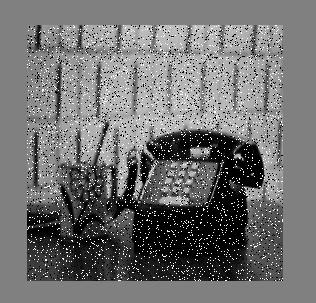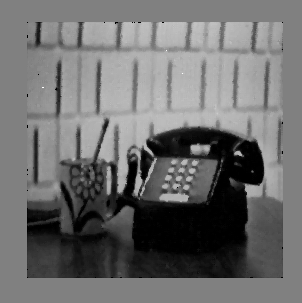GeeksforGeeks App
Open AppBrowser
Continue

# Noise removal using Median filter in C++

Median filtering is a nonlinear process useful in reducing impulsive, or salt-and-pepper noise. The median filter is also used to preserve edge properties while reducing the noise. Also, the smoothing techniques, like Gaussian blur is also used to reduce noise but it can’t preserve the edge properties. The median filter is widely used in digital image processing just because it preserves edge properties.

Approach :

• Store the pixel values of input image in an array.
• For each pixel value store all the neighbor pixel value including that cell in a new array (called window).
• Sort the window array.
• Median of window array is used to store output image pixel intensity.

Boundary Issues Example:
2D Median filtering example using a 3 x 3 sampling window:
Extending border values outside with values at the boundary.

Edge preservation :
All smoothing techniques are used to remove noise. The median filter is also one kind of smoothing technique like Gaussian filter, but the only difference between the median filter and Gaussian filter is that the median filter preserves edge property while Gaussian filter does not. Edge preservation is an important property because edges are important for visual appearance. For edge preservation property median filter is widely used in digital image processing.

C++ implementation of median filter algorithm.

## C++

 `#include ``#include ``#include ``using` `namespace` `std;` `/* Function to sort an array using insertion sort*/``void` `insertionSort(``int` `arr[], ``int` `n)``{``    ``int` `i, key, j;``    ``for` `(i = 1; i < n; i++)``    ``{``        ``key = arr[i];``        ``j = i - 1;` `        ``/* Move elements of arr[0..i-1], that are``        ``greater than key, to one position ahead``        ``of their current position */``        ``while` `(j >= 0 && arr[j] > key)``        ``{``            ``arr[j + 1] = arr[j];``            ``j = j - 1;``        ``}``        ``arr[j + 1] = key;``    ``}``}` `int` `array;``int` `arr;``int` `main()``{``    ``int` `window,row = 0, col = 0, numrows = 0, numcols = 0,MAX=0;``    ``ifstream infile(``"Saltpepper.pgm"``);``    ``stringstream ss;``    ``string inputLine = ``""``;` `    ``// First line : version``    ``getline(infile,inputLine);``    ``if``(inputLine.compare(``"P2"``) != 0) cerr << ``"Version error"` `<< endl;``    ``else` `cout << ``"Version : "` `<< inputLine << endl;` `    ``// Continue with a stringstream``    ``ss << infile.rdbuf();` `    ``// Secondline : size of image``    ``ss >> numcols >> numrows >> MAX;` `    ``//print total number of rows, columns and maximum intensity of image``    ``cout << numcols << ``" columns and "` `<< numrows << ``" rows"` `<> array[row][col];``        ``}``    ``}`  `    ``// Now print the array to see the result``    ``for``(row = 1; row <= numrows; ++row)``    ``{``        ``for``(col = 1; col <= numcols; ++col)``        ``{``            ``//neighbor pixel values are stored in window including this pixel``            ``window = array[row-1][col-1];``            ``window = array[row-1][col];``            ``window = array[row-1][col+1];``            ``window = array[row][col-1];``            ``window = array[row][col];``            ``window = array[row][col+1];``            ``window = array[row+1][col-1];``            ``window = array[row+1][col];``            ``window = array[row+1][col+1];` `            ``//sort window array``            ``insertionSort(window,9);``            ``//put the median to the new array``            ``arr[row][col]=window;   ``        ``}``    ``}` `    ``ofstream outfile;``    ` `    ``//new file open to store the output image``    ``outfile.open(``"Medianfilter.pnm"``);``    ``outfile<<``"P2"``<

Input ImageOutput ImageMy Personal Notes arrow_drop_up